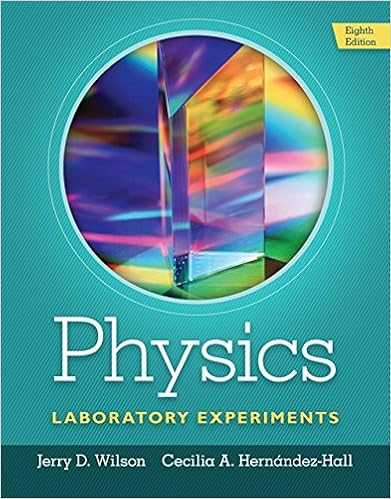# Experiment 2 - Vector Addition - Experiment 2 Pre-lab...

• Lab Report
• 5
• 83% (6) 5 out of 6 people found this document helpful

This preview shows page 1 - 3 out of 5 pages.

##### We have textbook solutions for you!
The document you are viewing contains questions related to this textbook.The document you are viewing contains questions related to this textbook.
Chapter 6 / Exercise 8
Physics Laboratory Experiments
WilsonExpert Verified
Experiment 2Pre-lab questions1) What is the basic difference between scalars and vectors?
2) Is temperature is a vector quantity? What do plus and minus temperature signify? Explain.3) A vector A has a magnitude of 60 m and directed as shown.Find the xand ycomponents of this vector.
4) What are, briefly, the steps to find the resultant of vectors?5) What is the difference between a resultant & equilibrant vector & what do they physically mean?
- 1 -
##### We have textbook solutions for you!
The document you are viewing contains questions related to this textbook.The document you are viewing contains questions related to this textbook.
Chapter 6 / Exercise 8
Physics Laboratory Experiments
WilsonExpert Verified
Experiment 2Objective1. Obtain the resultant of a number of forces acting on a body. 2. Obtain the resultant of the same forces by drawing a vector diagram to scale to find its magnitude and direction.3. Determine of the resultant using the analytical method, including the law of cosine for the magnitude and the
•••